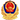# 2014年8月GMAT数学机经

2014-11-20 15:59:00 无忧考网
1. 有ABCDEFGHI 9个人，分到两个组中group x 和group y, 每个组最多不超过6人，求ABCEDF为一组，GHI为一组的概率。B: 1/210

步骤一：X、Y两组都不超过6人，则两组可能人数有四种组合：

组名： X Y

人数 ： 6 3 第一种组合

5 4 第二种组合

4 5 第三种组合

3 6 第四种组合

步骤二：XY人数分别为6、3时，可能情况为C39 =84(即X组里随便选6人，那剩下的3人当然就去了Y组);后面三种组合依次对应情况为126、126、84，四种共计420种情况;

步骤三：题目要求一组为指定的6人，另一组为剩下3人，只能出现在第一种和第四种组合中，只有两种情况，所以答案为2/420=1/210

2. V1：有个梯形(放置方式是斜边在上，上底在右下底在左)，上底是某个数字(失忆<180)，下底是180，高是300，然后以该梯形的高为长边有个矩形，矩形的宽就在梯形的上下底上，还给了一个面积条件(忘了是大梯形面积还是小梯形面积)，最后是求那个矩形的宽 (答案是30貌似)V2：梯形求边长，题目说得很复杂，修路神马的。整体上就是上面梯形侧过来，下面矩形，梯形阴影面积已知，两边长已知，求下面矩形宽度。(戳到图片了!) 设梯形上底x，下底y，巨星宽z

题目数据可能回忆不准确，我就不带入具体数据了，讲下思路：

梯形面积已知，高已知(两个狗主回忆的都是300)，所以上底加下底x+y=一个确定值

而x+z=某确定值(狗主回忆121)

y+z=某确定值(196?)

反正最后就是个三元一次方程组，肯定可以求出z

3. 一张矩形的纸，延一条线折了起来，顶点落在矩形的长边上，把长分为6和2， 是求矩形的长和折起来形成的那个大三角形的短边的比率(答案根号7)首先已知AE、BE，求得AB=2根7

三角形ABE相似与三角形ECF，所以AE/EF=AB/EC，

求得EF=8/根7

比例=BC/EF=根7

4. 一条数轴，等距分布A,B,C,D,E,F,G 其中E和F分别为3^11和3^12，求-3^12在哪个点?

数轴请自行脑补，这里不画了。

首先求出相邻两点的间距d=3^12 -3^11=3^11(3-1)=2*3^11;

以E点为标杆，要求的点(为负数)一定在E点左边，两点距离

D=3^11-(-3^12)=3^11+3^12=3^11(1+3)=4*3^11=2d;

所以差了两个间距，即向左两个间距，即C点。

5. 08*10的M次方 再 乘以 3.98*10的N次方 = 1398.09 * 10 的次方(这个次方告诉了数字，)

求T?

(数字是狗主乱编的，但是前两个数字的乘积是等式后面的开头。主要考的是10的次方的换算)

6. 好像N是什么15 18 40 50(好像还有一个数?我忘了)的最小公倍数，然后求N的取值范围，我就死算 算出来好像是1000多 然后选的是B 200≤N≤2000 (最小公倍数算法)

7. N>6 然后n!/【4!*(n-6)!】=(n-1)!/(n-5)! 算n等于多少 (可能(n-6)!和(n-5)!的位置有记差掉)

假如位置没有记错(具体什么位置考场上一定要亲手算，不能死记答案)

等式左边=[n(n-1)(n-2)(n-3)(n-4)(n-5)]/(4X3X2);

等式右边=(n-1)(n-2)(n-3)(n-4);

等式右边的全部消掉;

即n(n-5)/(4X3X2)=1，求得n=8

8. n=2^(x+y)*5^(xy)，能不能求出x和y?条件 (1)n=50 条件 (2)n=20

单独条件1：

50=21*52 有且只有这种情况(2,5组合的话)，

即x、y和为1，积为2，无解;

一定求不出，单独条件1对

单独条件2：

n=20=22*51

即x、y和为2，积为1;

求得x=y=1

单独条件2也可以，选D

9. 坐标系，A(-5,-10) B (-15 ,-10) ，能不能求出三角形ABC的面积 条件(1)点C在y=-1上 条件(2)点C是什么条件来着 忘了。(待补充)

10. x>=y?

条件(1) |x-y|=|x|-|y|

(2)|x-y|=x-y

单独条件1：

X=y=1,满足条件1，x大于等于y

X=-5，y=-3，满足条件1，但x小于y

不充分

单独条件2：

题干反过来下，x-y=|x-y|，后者一定大于等于0，所以x-y大于等于0，所以x大于等于y，充分;

答案：B

GMAT最新更新京公网安备 11010802026788号